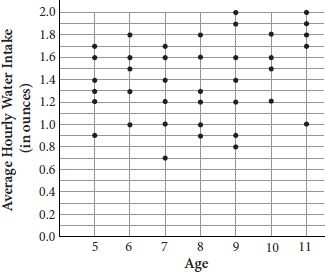# SAT Math Multiple Choice Question 854: Answer and Explanation

### Test Information

Question: 854

14. A dietician working in the children's ward at a hospital is monitoring the water intakeof all the patients in the ward. The total water intake for each patient is recordedthroughout the day and then averaged over the 24-hour period. The results are recordedin the scatterplot, where each dot represents the average hourly water intake forone child.Which of the following ages has the greatest range of values for the average hourlywater intake?

• A. Age 5
• B. Age 6
• C. Age 8
• D. Age 11

Explanation:

D

Difficulty: Medium

Category: Problem Solving and Data Analysis / Scatterplots

Strategic Advice: The range of a data set is the largest value minus the smallest value. In this question, you are comparing the ranges for each age, not for the whole data set.

Getting to the Answer: Read the axis labels carefully. Average hourly water intake is plotted along the vertical axis, and each grid-line represents 0.1. Estimate the y-coordinate of the lowest point and the highest point for each of the ages given in the answer choices. (Don't waste time calculating the ranges for all the ages plotted along the horizontal axis.) Then, subtract to find the range for each age:

Age 5: highest = 1.7; lowest = 0.9; difference = 0.8

Age 6: highest = 1.8; lowest = 1.0; difference = 0.8

Age 8: highest = 1.8; lowest = 0.9; difference = 0.9

Age 11: highest = 2.0; lowest = 1.0; difference = 1.0

The greatest difference is 1.0 (age 11), so (D) is correct.